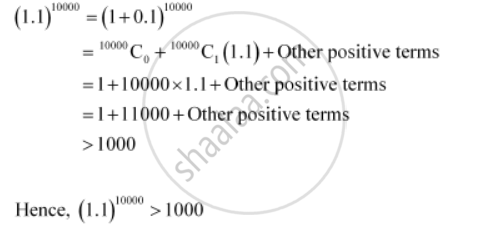CBSE (Arts) Class 11CBSE
Share
Notifications

View all notifications
Books Shortlist
Your shortlist is empty

# Using Binomial Theorem, Indicate Which Number is Larger (1.1)10000 Or 1000. - CBSE (Arts) Class 11 - Mathematics

Login
Create free account

Forgot password?
ConceptBinomial Theorem for Positive Integral Indices

#### Question

Using Binomial Theorem, indicate which number is larger (1.1)10000 or 1000.

#### Solution

By splitting 1.1 and then applying Binomial Theorem, the first few terms of (1.1)10000 can be obtained asIs there an error in this question or solution?

#### APPEARS IN

Solution Using Binomial Theorem, Indicate Which Number is Larger (1.1)10000 Or 1000. Concept: Binomial Theorem for Positive Integral Indices.
S